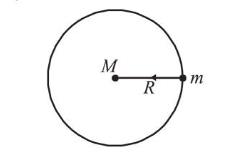# The mass density of a spherical galaxy varies as $rac{K}{r}$ over

Question:

The mass density of a spherical galaxy varies as $\frac{K}{r}$ over

a large distance ' $r$ ' from its centre. In that region, a small star is in a circular orbit of radius $R$. Then the period of revolution, $T$ depends on $R$ as :

1. (1) $T^{2} \propto R$

2. (2) $T^{2} \propto R^{3}$

3. (3) $T^{2} \propto \frac{1}{R^{3}}$

4. (4) $T \propto R$

Correct Option: 1,

Solution:

(1) According to question, mass density of a spherical

galaxy varies as $\frac{k}{r}$.

Mass, $M=\int_{0}^{r=R_{0}} \rho d V$

$\Rightarrow M=\int_{0}^{k} \frac{k}{r} 4 \pi r^{2} d r$

$\Rightarrow M=4 \pi k \int_{0}^{R_{0}} r d r$or, $M=\frac{4 \pi k R_{0}^{2}}{2}=2 \pi k R^{2}$

$F_{G}=\frac{G M m}{R_{0}^{2}}=m \omega_{0}^{2} R\left(=F_{C}\right)$

$\Rightarrow \frac{G \frac{4 \pi k R^{2}}{2}}{R^{2}}=\omega_{0}^{2} R \Rightarrow \omega_{0}=\sqrt{\frac{2 \pi K G}{R}} \quad\left(\because \omega=\frac{2 \pi}{T}\right)$

$\therefore T=\frac{2 \pi}{\omega_{0}}=\frac{2 \pi \sqrt{R}}{\sqrt{2 \pi K G}}=\sqrt{\frac{2 \pi R}{K G}} \Rightarrow T^{2}=\frac{2 \pi R}{K G}$

$\because 2 \pi, K$ and $G$ are constants

$\therefore T^{2} \propto R$